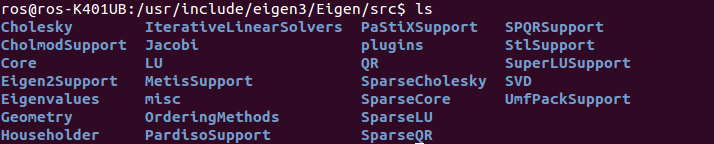# 深入學習 LSD-SLAM - 4

## 安裝與小玩一下 Eigen

sudo apt-get install libeigen3-devmkdir test_eigen
vim eigenMatrix.cpp

#include <iostream>
using namespace std;

#include <Eigen/Core>

int main( int argc, char** argv )
{
// Eigen 中所有向量和矩陣都是Eigen::Matrix。它的前三個參數分別是：資料行態，row 數，col 數
// 宣告一個 2x3 的 float 矩陣
Eigen::Matrix<float, 2, 3> matrix_23;

// 初始化 matrix 的 element
matrix_23 << 1, 2, 3, 4, 5, 6;
// 輸出
cout << matrix_23 << endl;

// 用 () 來存取 matrix 中的 element
for (int i=0; i<2; i++) {
for (int j=0; j<3; j++)
cout<<matrix_23(i,j)<<"\t";
cout<<endl;
}

return 0;
}


vim CMakeLists.txt


cmake_minimum_required( VERSION 2.8 )
project( eigenMatrix )

set( CMAKE_BUILD_TYPE "Release" )
set( CMAKE_CXX_FLAGS "-O3" )

include_directories( "/usr/include/eigen3" )

# in osx and brew install
# include_directories( /usr/local/Cellar/eigen/3.3.3/include/eigen3 )



cmake .
make
./eigenMatrix


ros@ros-K401UB:~/code/eigen\$ ./eigenMatrix
1 2 3
4 5 6
1 2 3
4 5 6


mkdir build
cd build
cmake ..
make


## 解線性方程組 & 座標轉換

#include <iostream>
#include <cmath>
using namespace std;

#include <Eigen/Core>
// Eigen/Geometry 提供了各种旋轉和平移的功能
#include <Eigen/Geometry>

int main ( int argc, char** argv )
{
// 3D 旋轉矩陣直接使用 Matrix3d 或 Matrix3f
Eigen::Matrix3d rotation_matrix = Eigen::Matrix3d::Identity();

// AngleAxis 就是旋轉向量,
//沿 Z 軸轉 45 度
Eigen::AngleAxisd rotation_vector ( M_PI/4, Eigen::Vector3d( 0,0,1 ) );
cout .precision(3);
//用matrix()轉成矩陣輸出
cout << "rotation matrix =\n" << rotation_vector.matrix() << "\n" << endl;

// 接著我們產生大家熟悉的旋轉矩陣
rotation_matrix = rotation_vector.toRotationMatrix();

// Eigen 使用 typedef 提供了許多容易理解的資料型態，但 Vector3d 其實只是 Eigen::Matrix<double, 3, 3>
Eigen::Vector3d v ( 1,0,0 );

// 用旋轉向量來旋轉
Eigen::Vector3d v_rotated = rotation_vector * v;
cout << "(1,0,0) after rotation = " << v_rotated.transpose() << "\n" << endl;

// 或者用旋轉矩陣旋轉
v_rotated = rotation_matrix * v;
cout << "(1,0,0) after rotation = " << v_rotated.transpose() << "\n" << endl;

// Euler angle: 可以從旋轉矩陣直接算出
Eigen::Vector3d euler_angles = rotation_matrix.eulerAngles ( 2,1,0 ); // roll pitch yaw
cout << "yaw pitch roll = " << euler_angles.transpose() << "\n" << endl;

// 四元數
// 可以直接把用 AngleAxis 來初始化
Eigen::Quaterniond q = Eigen::Quaterniond ( rotation_vector );
cout << "quaternion = \n" << q.coeffs() << "\n" << endl;   // 順序是(x,y,z,w)
// 也可以用旋轉矩陣來初始化
q = Eigen::Quaterniond ( rotation_matrix );
cout << "quaternion = \n" << q.coeffs() << "\n" << endl;
// 使用四元數來旋轉向量(應該要跟之前用旋轉向量或旋轉矩陣的結果一樣)
v_rotated = q*v;
cout << "(1,0,0) after rotation = " << v_rotated.transpose() << "\n" << endl;

return 0;
}


rotation matrix =
0.707    -0.707         0
0.707     0.707         0
0         0         1

(1,0,0) after rotation =    0.707    0.707        0

(1,0,0) after rotation =    0.707    0.707        0

yaw pitch roll = 0.785 -0  0

quaternion =
0
0
0.383
0.924

quaternion =
0
0
0.383
0.924

(1,0,0) after rotation =    0.707    0.707        0


## 延伸閱讀

@pojenlai 演算法工程師，對機器人跟電腦視覺有少許研究，最近在鍛鍊自己的執行力